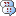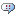##### Personal tools
You are here: Home Output units of the MATLAB scripts with hc-1 dataset

#Output units of the MATLAB scripts with hc-1 dataset

Up to Using data sets

### Output units of the MATLAB scripts with hc-1 datasetPosted by Marianne Case at April 17. 2012

Hello, I am using the MATLAB scripts to view the hc-1 dataset and want to understand the output units of the LoadBinary function.  I downloaded the data for d533101 and called the loadBinary function to read it into MATLAB:

function [data OrigIndex]= LoadBinary(FileName, Channels, varargin)

I only wanted the intracellular trace which the README.txt says is the 6th channel, so my call looked like:

[data OrigIndex]= LoadBinary('./buzsaki_data/d5331/d533101.dat', 6);

I received an error because the function 'datatypesize' was undefined; I created one myself that returns the size in bytes of the data type passed in as a string.

The resulting output resembles an intracellular trace, but I'm unsure of the units. For the x-axis, I assume it is just sample number; the README.txt gives the sampling rate (10 kHz) so I can get the time easily.  For the y-axis, the y values range from 1114 to 2193. I don't know what those values mean nor how to convert them into mV?

### Re: Output units of the MATLAB scripts with hc-1 datasetPosted by Jeff Teeters at April 19. 2012

I forwarded this question to Anton Sirota.  His response follows:

Binary data are stored, depending on the dataset, as 12 or 16bit digitized voltage at 10 or 20 kHz sampling rate.
File coding is 2byes (short int) for each digitized sample: Sample1_Channel1 Sample1_Channel2 ... Sample1_ChannelN Sample2_Channel1 ...
LoadBinary is my routine to load such binary file into a matrix: nLoadedChannels x nLoadedSamples
Better call is passing channels you want to load :

data = LoadBinary('./buzsaki_data/d5331/d533101.dat', 6, 6);

sampling rate should be indicated in par file , use LoadPar to see the values (or look into this ASCII file)
voltage - look into data description (I think there was one) for the amplification
to convert you need to divide digital range (2^15 or 2^16 by the voltage range of the amplifier divided by the gain). this will give you scaling factor,  something like this works, I think for this dataset too:

Par = LoadPar([FileBase '.xml']);
if Par.nBits==16
Vm = data./2^15*200;
elseif Par.nBits==12
Vm = (data-Par.Offset)./2^11*200;
end
best
Anton

### Re: Output units of the MATLAB scripts with hc-1 datasetPosted by Marianne Case at April 19. 2012

Thanks Jeff and Anton! I was able to convert the data, but I wanted to just add a few follow up notes here for other forum readers.

Regarding the LoadBinary call you suggested:

data = LoadBinary('./buzsaki_data/d5331/d533101.dat', 6, 6);

The third argument is the total number of channels, nChannels, which is not 6 - I get an error if I add in that third argument.  The second argument is the specific channel I want, #6, so only calling the function with 2 arguments works just fine and is quick.

I tried the LoadPar function to check on the par values as you suggested. Regarding the par file comments:

sampling rate should be indicated in par file

Indeed, it was and it matched the value in the README.txt, 10000.  However, not all the values in the .xml Par file made sense:

SampleTime is listed as 100, but the data vector covers 240 s.  Voltage Range is listed as 20 and Amplification as 1000, but in the dataset description file the "voltage amplication" is listed as 50. That makes sense if the voltage amplification is defined as amplification/voltage range, but I don't know the relation between the terms in the Par file "Voltage Range", "Amplification" and the terms in your post "voltage amplification", "gain."

The par file also gives nBits = 16.  However, when I used the conversion listed above for nBits = 16:

Vm = data./2^15*200;

I get a voltage trace that is not realistic.  I used the conversion for nBits = 12:

Vm = (data-Par.Offset)./2^11*200;

and got a realistic trace (meaning AP threshold, AP amplitude, and resting membrane potential are realistic/within usual physiological range).

### Re: Output units of the MATLAB scripts with hc-1 datasetPosted by Sirenia Lizbeth at September 22. 2015

Hello,

This question has been posted a while ago but it seems to me that the answer is not clear enough.

I am using the MATLAB scripts to view the hc-2 dataset (file ec013.529) and I  followed the instructions for hc-1 conversion.

For ec013.529 file the LoadPar function returns the following:

nBits: 16                       SampleRate: 20000                      SampleTime: 50

VoltageRange: 20     Amplification: 1000                      Offset: 0

So according to this parameters the conversion should be something like:

Vm = data./2^15*VoltageRange;      But seeing the traces I am not sure that the y-axis values are correct.

For dataset hc-1 file d533101.dat (original question posted in 2012) the parameters are

nBits: 16                      SampleRate: 10000                      SampleTime: 100

VoltageRange: 20    Amplification: 1000                      Offset: 2048

But the solution given was

Vm = (data-Offset)./2^11*200;

My questions are

-Why is 200 used as system voltage range if in the parameters is listed as 20?

-Were xml data description files always verified?

Thank you!

### Re: Output units of the MATLAB scripts with hc-1 datasetPosted by Geoffrey Barrett at October 19. 2018

Yes this is quite confusing. The HC-1 data description (https://crcns.org/files/data/hc-1/crcns-hc1-data-description.pdf) says that the gain/amplification for the IC is typically 50 and the EC is typically 1000. However, the .xml only mentions a gain of 1000, so does that mean that both the EC and IC have that gain? Or is the 1000 value just representative of the EC data?

Also is the voltage range 20V? Is there a different voltage range for the IC data?

The conversion from bits to volts should be X = Voltage Range / (16 bit range * gain), where 16 bit range is 2^16, and you can multiply Data * X to get the voltage. I suppose you have to remove the offset that was mentioned so it would be (data-offset)*X. However you might have two X's depending on if there are varying voltage ranges and gains for the EC and IC data.

### Re: Output units of the MATLAB scripts with hc-1 datasetPosted by abolfazl rahimi at November 23. 2018

thanks helped me a lot

Powered by
##### Document Actions